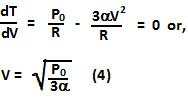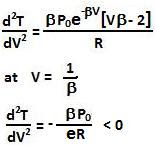## Monday, January 25, 2010

### Irodov Problem 2.11

Since its one mole of an ideal gas we have,a) Also since we know that,Hence we have,Extrema of the temperature will occur when the first derivative is zero.The second derivative is given by,Hence this corresponds to a maximum. So we have,b) Similar to above, using ideal gas equation and given relationship between P and V the we have,Extrema of the temperature will occur when the first derivative is zero.The second derivative is given by,Hence, this corresponds to a maximum. So from (5) and (6) we have,1.this is a bit difficult way to solve q-11.11
can't it be solved by an easier method.

2.no i think this is good way to understand problem

3.The Gioco digitale domain stillcasino.com | Updated 2021 - New Games & More
The Gioco digitale is the number one casino game for slots players. This casino uses a chip 메리트카지노 of the gioco digitale iSoftBet chip which is 1XBET used in the design to